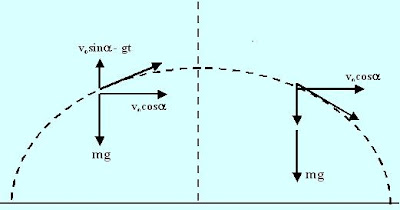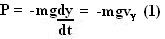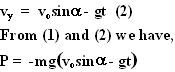## Saturday, February 16, 2008

### Irodov Problem 1.125The work done by gravity when the body moves a upward vertical distance dy is given by -mgdy (since displacement is opposite to direction of gravity) . The instantaneous power is the derivative of work done and is given by,a) For the first part the answer is simply 0. This is because before reaching the halfway point the power developed by gravity is always negative (since velocity is upwards and force is downwards). After the halfway point the power generated by gravity is always positive since the body starts to move in the same direction as the force of gravity. For every point A in the left half of the path, there exists a symmetric point A' on the right half of the path where the vertical velocity of body at A is exactly equal and opposite to that at A' so that sum of the instantaneous powers at A and A' will be 0. This means that the negative power accumulated over the first half of the path will be exactly canceled out by the positive power accumulated by the second half of the path.

b) At any instant of time t the vertical velocity of the body is given by (see here for more about projectile motion),